# Isoperimetric inequality, classical

The inequality between the volumeof a domain in a Euclidean space,, and the-dimensional areaof the hypersurface bounding the domain: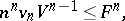whereis the volume of the unit-sphere. Equality holds only for a sphere. The classical isoperimetric inequality gives a solution of the isoperimetric problem. Forthe classical isoperimetric inequality was known in Antiquity. A rigorous proof of the classical isoperimetric inequality forwas given by F. Edler in 1882, for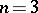by H.A. Schwarz in 1890, and for allby L.A. Lyusternik in 1935 and E. Schmidt in 1939 (see , , ).

While in the two-dimensional case there are many proofs of the classical isoperimetric inequality (see ), only two approaches are known for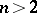. The first is the method of symmetrization proposed by J. Steiner. Using this method, Schmidt obtained analogues of the classical isoperimetric inequality (and the Brunn–Minkowski inequalities) for spherical and hyperbolic-dimensional spaces (see ). The second approach consists in reducing the classical isoperimetric inequality to a Brunn–Minkowski inequality (see Brunn–Minkowski theorem) and using the method of proportional division of volumes. In this approach there naturally arises the more general inequality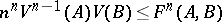(*)

for volumes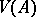,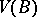of two sets and the Minkowski areaof the setwith respect to. The inequality (*) can be interpreted as a classical isoperimetric inequality in Minkowski space; equality for a fixed Minkowski "sphere"is not, generally speaking, attained for a unique body; moreover, these bodies are different from a "sphere" (see ).

There are a number of generalizations of the classical isoperimetric inequality in which one does not consider domains with a piecewise-smooth boundary, but wider classes of sets, and the area of the boundary is considered in a generalized sense (Minkowski area, Lebesgue area, Caccioppoli–De Giorgi perimeter of a set, or the mass of a current, see , ). The classical isoperimetric inequality remains valid in all these cases, as well as for hypersurfaces with self-intersections and the corresponding oriented volume (see ). These generalizations can be obtained from the classical isoperimetric inequality by limit transition for distinct variants of the concept of convergence.

For the isoperimetric difference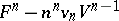, and the isoperimetric ratio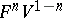, estimates are known which strengthen the classical isoperimetric inequality (see ). Some of these estimates are obtained for sets of special shape, in the first place for convex sets (cf. Convex set) and polyhedra (see ). An example of this is the Bonnesen inequality for plane figures: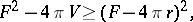whereis the radius of the largest inscribed circle, and its generalization (see ) for convex bodies in: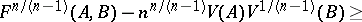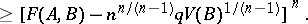Here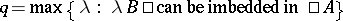. The relative isoperimetric difference of two convex bodies,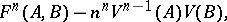can serve as a measure of their non-homotheticity (see ). It is used, for example, in proving stability theorems in the Minkowski problem (see ). For generalizations of the classical isoperimetric inequality to spaces of variable curvature and related inequalities, see Isoperimetric inequality.